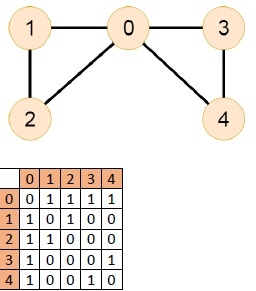# C++ Program to Check Whether an Undirected Graph Contains a Eulerian Cycle

To know about Euler Circuit, we have the idea about Euler Path. The Euler path is a path; by which we can visit every node exactly once. We can use the same edges for multiple times. The Euler Circuit is a special type of Euler path. When the starting vertex of the Euler path is also connected with the ending vertex of that path.

To detect the circuit, we have to follow these conditions:

• The graph must be connected.
• Now when no vertices of an undirected graph have odd degree, then it is a Euler Circuit.

## Input## Output

The graph has Euler Circuit.

## traverse(u, visited)

Input The start node u and the visited node to mark which node is visited.

Output Traverse all connected vertices.

Begin
mark u as visited
for all vertex v, if it is adjacent with u, do
if v is not visited, then
traverse(v, visited)
done
End

### isConnected(graph)

Input : The graph.

Output : True if the graph is connected.

Begin
define visited array
for all vertices u in the graph, do
make all nodes unvisited
traverse(u, visited)
if any unvisited node is still remaining, then
return false
done
return true
End

## hasEulerianCircuit(Graph)

Input The given Graph.

Output Returns 0, when no Eulerian Circuit, and returns 1 when it has Euler circuit..

Begin
if isConnected() is false, then
return false
define list of degree for each node
oddDegree := 0
for all vertex i in the graph, do
for all vertex j which are connected with i, do
increase degree
done
if degree of vertex i is odd, then
increase oddDegree
done
if oddDegree is 0, then
return 1
else return 0
End

## Example Code

#include<iostream>
#include<vector>
#define NODE 5
using namespace std;
/*int graph[NODE][NODE] = {{0, 1, 1, 1, 0},
{1, 0, 1, 0, 0},
{1, 1, 0, 0, 0},
{1, 0, 0, 0, 1},
{0, 0, 0, 1, 0}};*/ //No Euler circuit, but euler path is present
int graph[NODE][NODE] = {{0, 1, 1, 1, 1},
{1, 0, 1, 0, 0},
{1, 1, 0, 0, 0},
{1, 0, 0, 0, 1},
{1, 0, 0, 1, 0}}; //uncomment to check Euler Circuit as well as path
/*int graph[NODE][NODE] = {{0, 1, 1, 1, 0},
{1, 0, 1, 1, 0},
{1, 1, 0, 0, 0},
{1, 1, 0, 0, 1},
{0, 0, 0, 1, 0}};*/ //Uncomment to check Non Eulerian Graph
void traverse(int u, bool visited[]) {
visited[u] = true; //mark v as visited
for(int v = 0; v<NODE; v++) {
if(graph[u][v]) {
if(!visited[v]) traverse(v, visited);
}
}
}
bool isConnected() {
bool *vis = new bool[NODE];
//for all vertex u as start point, check whether all nodes are visible or not
for(int u; u < NODE; u++) {
for(int i = 0; i<NODE; i++)
vis[i] = false; //initialize as no node is visited
traverse(u, vis);
for(int i = 0; i<NODE; i++) {
if(!vis[i]) //if there is a node, not visited by traversal, graph is not connected
return false;
}
}
return true;
}
int hasEulerianCircuit() {
if(isConnected() == false) //when graph is not connected
return 0;
vector<int> degree(NODE, 0);
int oddDegree = 0;
for(int i = 0; i<NODE; i++) {
for(int j = 0; j<NODE; j++) {
if(graph[i][j])
degree[i]++; //increase degree, when connected edge found
}
if(degree[i] % 2 != 0) //when degree of vertices are odd
oddDegree++; //count odd degree vertices
}
if(oddDegree == 0) { //when oddDegree is 0, it is Euler circuit
return 1;
}
return 0;
}
int main() {
if(hasEulerianCircuit()) {
cout << "The graph has Eulerian Circuit." << endl;
} else {
cout << "The graph has No Eulerian Circuit." << endl;
}
}

## Output

The graph has Eulerian Circuit.